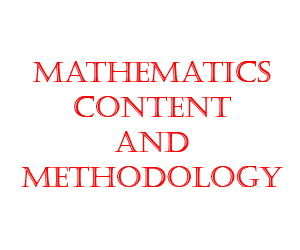# Mathematics TET Paper 2 Free Online Mock TestMathematics TET Paper 2 Free Online Mock Test

TRT TET Paper 1 MATHEMATICS

Mathematics TET Paper 2 mcq questions are very important objective multiple choice questions for crack TET Paper 2 in Telangana, Andhra Pradesh based on D.Ed, B.Ed books. This Mathematics TET Paper 2 Practice Test is very useful for students to get good score in TET, TRT examination.

Mathematics TET Paper 2 Free Online Mock Test is useful fro crack TS TET, AP TET, CTET, UP TET, KTET etcMathematics TET Paper 2 Syllabus For TET, TRT:
(Marks: 30)
CONTENT (Marks: 24)

1. Number System –
Prime and Composite Numbers, Tests of divisibility, whole numbers, integers, fractions,
decimal fractions, L.C.M. and G.C.D. rational numbers and irrational numbers. Properties of numbers, Real
numbers; laws of exponents, squares, square roots, cubes, cube roots, finding missing number represented
as alphabets in sums involving array of four operations, number patterns, number puzzles, Euclid division
lemma, concept of logarithms.

2. Arithmetic –
Ratio and proportion, simple interest, compound interest, Time and distance, Discount, tax, time and work, profit and loss

3. Sets –
Concept of set, set language, empty set, finite and infinite sets, subset and equality of sets, cardinal
number of set, set operations, representation of sets, Venn diagrams and their properties,

4. Algebra –
Introduction to Algebra, expressions, exponents and powers, Factorization special products and expansions, linear equations and their graphs, polynomials, Quadratic equations and its applications, concept of progressions, progressions (AP and GP).nth term and sum of the first n terms in AP, nth term of GP

5. Geometry –
History of Geometry, Contribution of India in the Development of Geometry, Euclid Geometry, Lines and Angles, Similar Triangles, Pythagoras theorem, congruency of triangles, Properties of Circles, Triangles, Quadrilaterals and polygons, Parts of Circle : Construction of Circle, Triangles and Quadrilaterals, Circles and concurrent lines in triangles, Co-ordinate Geometry, Co-ordinates of a point, plotting of points, representing of linear equations in two variables (of the form ax+by+c=0, ) in the Cartisian coordination system). Linear equations with 2 variables, slope of a line, distance between two points in a plane, section formula, Area of a triangle, collinearity of points in a co-ordinate plane, centroid of a triangle, symmetry,

6. Mensuration –
Perimeter and Area of a Square and Rectangle. Area of Triangle, Circle, Ring and Quadrilaterals. Surface area and volume of Cube, Cuboid, Lateral & total Surface area and volume of a cylinder, cone, sphere and hemisphere, conversion of one solid to another shape, surface area and volume of combination of solids.

7. Data Handling –
Collection and Classification of Data, Frequency distribution table, Tally marks, Bar graph, Pictograph and Pie diagrams, mean, median and mode for un-grouped and grouped data, cumulative frequency table and ogive curves, concept of probability, simple problems (day to day life situation) on single events, concept of complementary events.

8. Trigonometry –
Introduction of Trigonometry, Relationship between ratios, values of trigonometric ratios,
(0°, 30°, 45°, 60° and 90°) Trigonometric identities, trigonometric ratios of complementary angles,
applications of trigonometry, Angle of elevation and depression, simple problems.

Mathematics PEDAGOGY (Marks: 06)
1. Definitions and Nature of Mathematics
2. Aims, values , instructional objectives of teaching Mathematics and Academic Standards
3. Methods of Teaching Mathematics
4. Instructional material in Mathematics – TLM in Mathematics
5. Instructional Planning
6. Continuous Comprehensive Evaluation (CCE) – Formative Assessment, Summative
Assessment – Processes and procedures
7. Designing, Administration, Analysis of scholastic Achievement test (SAT)
8. Diagnostic and Remedial Teaching
9. The Mathematics Teacher
10. Resource Utilization
11. Curriculum and Text Book

Total Questions:24
Marks: 24
Negative Marks: NO
Durations: 24 Minutes

## Leaderboard: TRT TET Paper 1 MATHEMATICS

maximum of 118 points
Pos. Name Entered on Points Result